Test: Fluid Mechanics Level - 4

# Test: Fluid Mechanics Level - 4

Test Description

## 25 Questions MCQ Test Mechanical Engineering SSC JE (Technical) | Test: Fluid Mechanics Level - 4

Test: Fluid Mechanics Level - 4 for Mechanical Engineering 2022 is part of Mechanical Engineering SSC JE (Technical) preparation. The Test: Fluid Mechanics Level - 4 questions and answers have been prepared according to the Mechanical Engineering exam syllabus.The Test: Fluid Mechanics Level - 4 MCQs are made for Mechanical Engineering 2022 Exam. Find important definitions, questions, notes, meanings, examples, exercises, MCQs and online tests for Test: Fluid Mechanics Level - 4 below.
Solutions of Test: Fluid Mechanics Level - 4 questions in English are available as part of our Mechanical Engineering SSC JE (Technical) for Mechanical Engineering & Test: Fluid Mechanics Level - 4 solutions in Hindi for Mechanical Engineering SSC JE (Technical) course. Download more important topics, notes, lectures and mock test series for Mechanical Engineering Exam by signing up for free. Attempt Test: Fluid Mechanics Level - 4 | 25 questions in 50 minutes | Mock test for Mechanical Engineering preparation | Free important questions MCQ to study Mechanical Engineering SSC JE (Technical) for Mechanical Engineering Exam | Download free PDF with solutions
 1 Crore+ students have signed up on EduRev. Have you?
Test: Fluid Mechanics Level - 4 - Question 1

### When a block of ice floating on water in a container melts, the level of water in the container

Test: Fluid Mechanics Level - 4 - Question 2

### The body whose surface does not coincide with the streamline when places in a flow is called as

Test: Fluid Mechanics Level - 4 - Question 3

### A large metacentric height in a vessel

Test: Fluid Mechanics Level - 4 - Question 4

The time of oscillation of a floating body is given by Where
K = Radius of Gyration of the floating body about its centre of gravity
h = Metacentric height of the floating body

Test: Fluid Mechanics Level - 4 - Question 5

A cylindrical body of cross-sectional area A height H and densityrs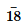is immersed to a depth h in a liquid of density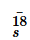r and tied down to bottom with a string then the tension in the string is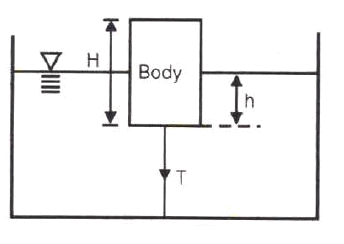Test: Fluid Mechanics Level - 4 - Question 6

There is no geometrical distinction between the streamline, pathline and streak line in case of

Test: Fluid Mechanics Level - 4 - Question 7

Stream line, path line and streak line are identical when the

Test: Fluid Mechanics Level - 4 - Question 8

Match List I with List II and select the correct answer using the codes given below the lists: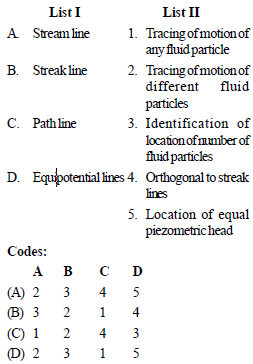Test: Fluid Mechanics Level - 4 - Question 9

The continuity equation in differential form is

Test: Fluid Mechanics Level - 4 - Question 10

Under which of the following conditions will the equation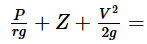constant, will be valid in the whole flow field ?

1. Flow is rotational
2. Flow is irrotational
3. Flow is incompressible
5. Flow is laminar

Test: Fluid Mechanics Level - 4 - Question 11

Venturimeter consists of following parts :
1. Diverging part
2. Throat
3. Main inlet pipe
4. Short converging part
Select the correct sequence of parts

Test: Fluid Mechanics Level - 4 - Question 12

The range for coefficient of discharge (Cd) for a venturimeter is

Test: Fluid Mechanics Level - 4 - Question 13

In the siphon in figure below assuming ideal flow, the pressure PB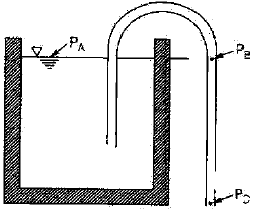Test: Fluid Mechanics Level - 4 - Question 14

The flow of fluid through a pipe is laminar when

Test: Fluid Mechanics Level - 4 - Question 15

An oil of kinematic viscosity 0.5 stokes flows through a pipe of 4 cm diameter. The flow is critical at a velocity of about

Test: Fluid Mechanics Level - 4 - Question 16

In the Navier-Stokes equations, the forces considered are

Test: Fluid Mechanics Level - 4 - Question 17

Navier-Stokes equation represents the conservation of

Detailed Solution for Test: Fluid Mechanics Level - 4 - Question 17

The Navier-Stokes equations govern the motion of fluids and can be seen as Newton's second law of motion for fluids. ... The Navier-Stokes equations represent the conservation of momentum, while the continuity equation represents the conservation of mass.

Test: Fluid Mechanics Level - 4 - Question 18

For laminar flow between two fixed parallel plates, the flow velocity

Test: Fluid Mechanics Level - 4 - Question 19

Capillary tube viscometers used for measurement of viscosity are based on

Test: Fluid Mechanics Level - 4 - Question 20

An incompressible fluid (kinematic viscosity, 7.4 × 10–7 m2/s, specific gravity, 8.88) is held between two parallel plates. If the top plate is moved with a velocity of 0.5 m/s while the bottom one is held stationary, the fluid attains a linear velocity profile in the gap of 0.5 mm between these plates; the shear stress in Pascals on the surface of top plate is

Test: Fluid Mechanics Level - 4 - Question 21

Pressure drop in a 100 mm diameter horizontal pipe is 50 kPa over a length of 10 m. The shear stress at the pipe wall is

Test: Fluid Mechanics Level - 4 - Question 22

The pressure drop for a relatively low Reynolds number flow in a 600 mm, 30 m long pipe line is 70 kPa. What is the wall shear stress

Test: Fluid Mechanics Level - 4 - Question 23

When the hydrodynamic boundary layer thickness (δ), which is the distance from the surface at which the local velocity (u) reaches 99% of the free-stream velocity, then

Test: Fluid Mechanics Level - 4 - Question 24

Shear velocity is

Test: Fluid Mechanics Level - 4 - Question 25

The head loss in a sudden expansion from 5 cm diameter to 10 cm diameter pipe, in terms of velocity V1 in the 5 cm pipe

## Mechanical Engineering SSC JE (Technical)

6 videos|89 docs|55 tests
 Use Code STAYHOME200 and get INR 200 additional OFF Use Coupon Code
Information about Test: Fluid Mechanics Level - 4 Page
In this test you can find the Exam questions for Test: Fluid Mechanics Level - 4 solved & explained in the simplest way possible. Besides giving Questions and answers for Test: Fluid Mechanics Level - 4, EduRev gives you an ample number of Online tests for practice

## Mechanical Engineering SSC JE (Technical)

6 videos|89 docs|55 tests